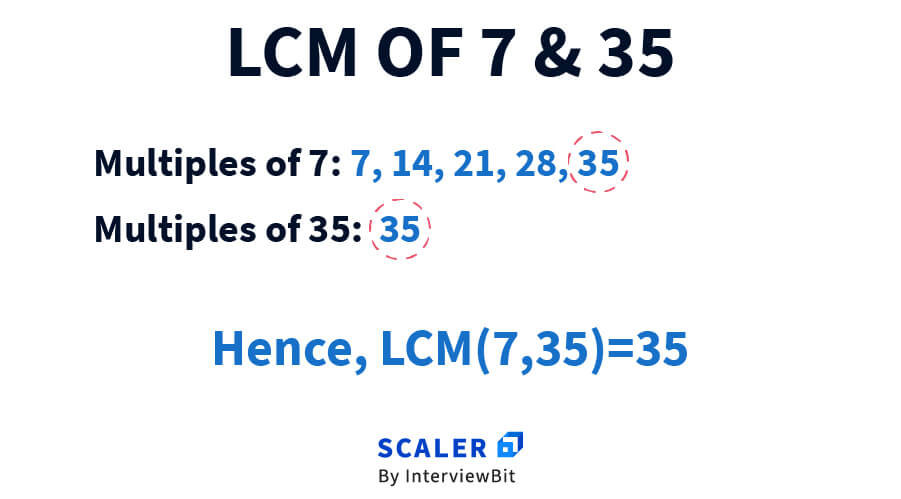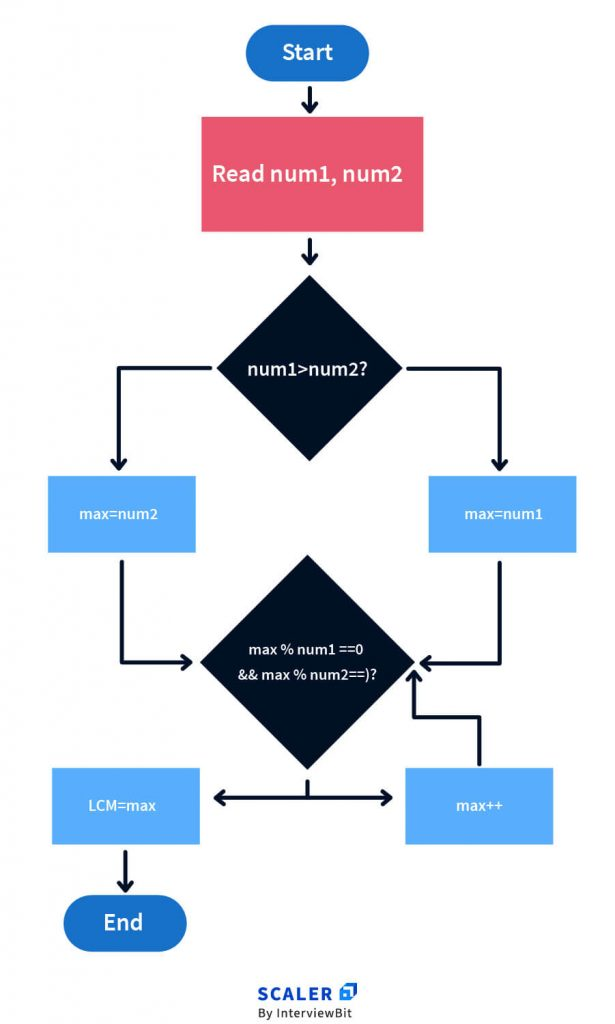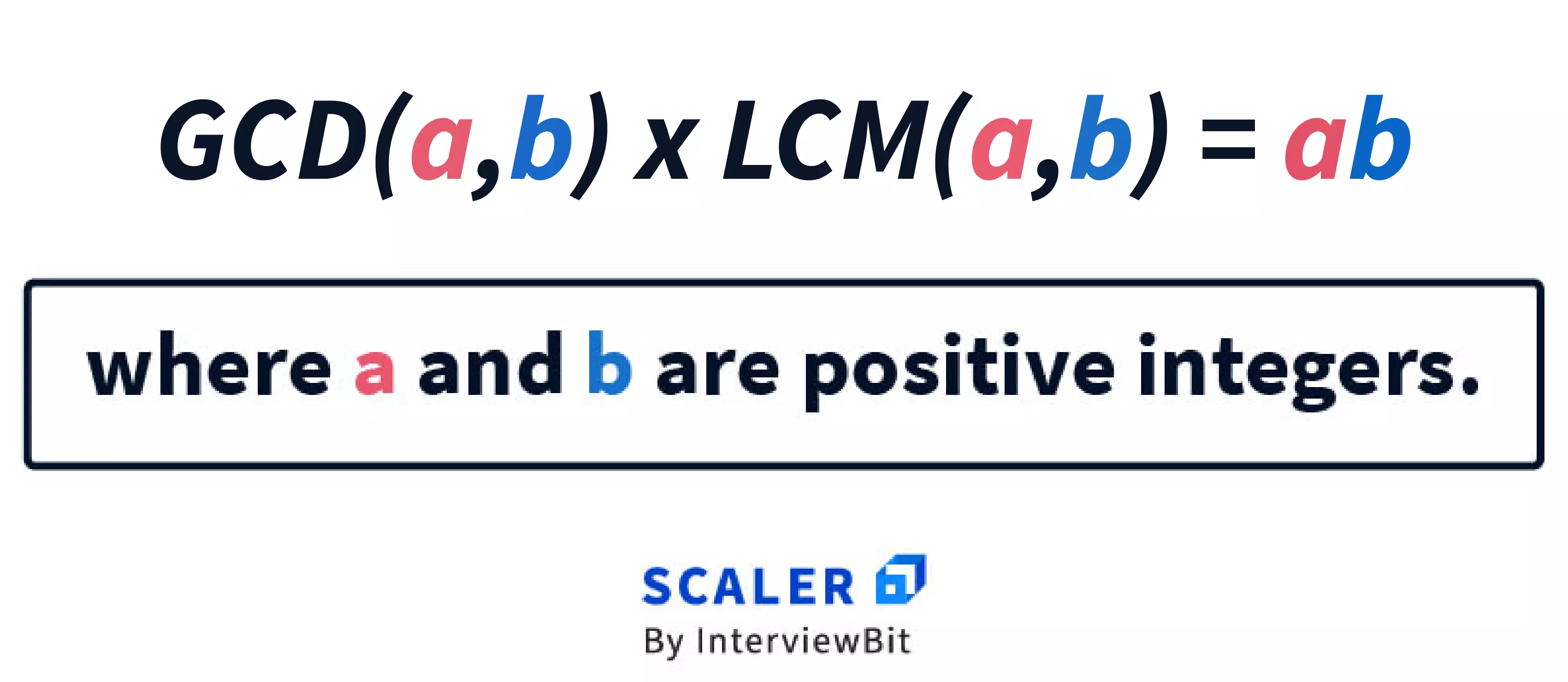# Program to Find LCM of Two Numbers in C

Challenge Inside! : Find out where you stand! Try quiz, solve problems & win rewards!
Learn via video courseC++ Course: Learn the Essentials
By Prateek NarangFree
5Enrolled: 1000C++ Course: Learn the Essentials
Prateek NarangFree
5
Enrolled: 1000

## Overview

The LCM program in C is a very common, beginner-level question asked. Before we dive deeper, we must understand what LCM is. We have all learned GCD(HCF) and LCM in school, however, we often forget what it is and when they are used.

Let us take a look at LCM in detail now.

## What is LCM?

To find the LCM of 2 numbers, you find all multiples of both numbers and find the smallest common multiple that the two numbers share. If we have two numbers, 7 (num1) and 35 (num2), and since we know that 35 is a multiple of 7, we can conclude that 35 is the LCM.

These are the real-life applications of LCM:

1. When we must buy or obtain several items so as to have enough.
2. When we need to know if something will happen once more at the same time.
3. When we must find out if an event will be repeated.

For example, Person A has a holiday once in 1 month while Person B has a holiday once in 1.5 months. If Person A and Person B both had a holiday today, how many days later will they both have a holiday together again?

An Example is Shown Below:

The smallest multiple the two numbers share is 35 and hence that is the LCM. A simple way to remember this is that the lowest common multiple is a number larger than or equal to the numbers in question, while the greatest common divisor is a number smaller than or equal to the numbers in question.## Algorithm

To find the LCM of two numbers in C, we will assume the two numbers are num1 and num2 and that they are positive integers. We will use the following approach:

START

Step 1: Initialize two variables for num1(7) and num2(35).

Step 2: Find and store the maximum of num1 and num2 to a separate variable, ‘max’(35).

Step 3: If max is divisible by num1(35 % 7 == 0?) and num2(35 % 35 == 0?), max is the LCM(35), hence print it.

Step 4: If not divisible, then increment max by 1, and go to step 3 until a number has been printed. Repeat the process of 3->4->3 until a max value is found that satisfies the constraints.

STOP## 4 Ways for Writing LCM Program in C

There are 4 ways in which the LCM Program in C can be written.

### a. While loop

Now that we have studied the algorithm let us implement the same using a while loop.

Explanation :

The following code assigns to the variable ‘max’ the number that’s the largest between num1 and num2 using the ternary operator.

A ternary operator has been used in the above line, which has the syntax of condition.

value_if_true : value_if_false.

while(1) is an infinite loop until it comes across an explicit break statement and it is used to find the LCM of two numbers in C as we must repeatedly check whether or not max is divisible by the two numbers. Once such a number is found, we print the number and break from the loop.

Code:

Output :

### Time Complexity:

O(X), where x = num1 * num2 Our while loop runs till res reaches LCM, i.e. 35.

For example, in this case both 7 and 5 are prime numbers, hence LCM will be the product of the two numbers, therefore loop will run till 35, and stop there as it suits all criteria, hence the time complexity is O(x), where x = num1 * num2.

### b. Function

In order for code to be reused, we can put the while loop in a function and call it so as to find the LCM.

Explanation:

The same algorithm is followed for the while loop as in the LCM program in C using while, except this time, we have a function call as:

Functions have the following advantages –

1. They increase the readability of code.
2. They improve code reusability; rather than developing the same code from scratch, the same function can be used in any program.
3. Using functions makes debugging the code easier because faults are more easily traced.

Code:

Output:

### Time Complexity:

O(X), where x = num1 * num2

Our while loop runs till res reaches LCM, i.e. 35.

For example, in this case both 7 and 5 are prime numbers, hence LCM will be the product of the two numbers, therefore loop will run till 35, and stop there as it suits all criteria, hence the time complexity is O(x) where x = num1 * num2.

### c. Recursive Function

A recursive function is a routine that calls itself explicitly or indirectly in programming terms. Certain problems can be solved quickly using the recursive algorithm.

Explanation:

The same algorithm is followed for the if block as in the lcm program in c using while, except this time, instead of using an infinite while loop, we have a function call as displayed below:

This function is called again in the else block if the lcm is not found and hence, it keeps running until an lcm is found which is why we do not require an infinite while loop in the lcm program in c using functions.

Code:

Output:

### d. GCD

The relationship between GCD and LCM is as illustrated below:

Explanation:

To find LCM of two numbers in C:

• Ask user to enter two variables.
• Using a for loop, find a number, starting from 1 that’s smaller than num1 and num2 that satisfies the condition: num1 % i == 0 and num2 % i == 0.
• Largest ‘i’ in the for loop that suits the above mentioned criteria, is the GCD.
• Find LCM by using the formula given, i.e. $\frac{(num1*num2)}{gcd}$ .Code:

Output:

### Time Complexity:

Here the loop runs till the minimum of both the numbers of the input and we find the greatest common factor for both the numbers and store it in a variable called gcd and hence worst-case time complexity = O(min(num1,num2)).

### e. GCD Optimized

We can also use Euclid's algorithm to calculate the GCD of two numbers in an optimized manner.

Code:

Output:

### Time Complexity:

Since we are using Euclid's algorithm to find the GCD of two numbers, the final time complexity is O(log(max(num1, num2))).

## Conclusion

• In this article, you have learned 4 ways in which you can find the LCM of two numbers in C.
• While it may not be asked directly by companies in their recruitment drives, you might find yourself having to calculate LCM while solving programming questions that have a higher difficulty level.
• Moreover, knowing the logic behind finding the LCM could also be fruitful for aptitude questions asked in the recruitment process.